# Python module

This page shows some simple examples of using the `planetmapper` module in Python code. For more details, see the full API documentation.

## Coordinate conversions

Coordinate conversions can easily be performed using functions such as `planetmapper.Body.lonlat2radec()` to calculate the sky coordinates corresponding to a planetographic longitude/latitude coordinate on the surface of the target.

This code shows an example of using some of the functions in `planetmapper.Body` to calculate information about observations of Jupiter from Mars:

```import planetmapper
import numpy as np
import matplotlib.pyplot as plt

body = planetmapper.Body('jupiter', '2020-01-01', observer='mars')

coordinates = [(42, 0), (123, 45)]
for lon, lat in coordinates:
print(f'\nlongitude = {lon}°, latitude = {lat}°')
if body.test_if_lonlat_visible(lon, lat):
print(f'  RA = {ra:.4f}°, Dec = {dec:.4f}°')
if body.test_if_lonlat_illuminated(lon, lat):
phase, incidence, emission = body.illumination_angles_from_lonlat(lon, lat)
print(f'  phase angle: {phase:.2f}°')
print(f'  incidence angle: {phase:.2f}°')
print(f'  emission angle: {phase:.2f}°')
else:
print('  (Not visible)')
```

Hint

The main classes in PlanetMapper are subclasses of each other, with `planetmapper.SpiceBase` the parent class of `planetmapper.Body` which is the parent of `planetmapper.BodyXY` which is the parent of `planetmapper.Observation`.

In Python, any functions defined in a parent class are available in any subclasses, so for example, you can use `planetmapper.Observation.lonlat2radec()` exactly the same way as you can use `planetmapper.Body.lonlat2radec()`.

## Wireframe plots

‘Wireframe’ plots showing the geometry of target bodies can be created quickly and easily using the `planetmapper.Body.plot_wireframe_radec()` command:

```body = planetmapper.Body('saturn', '2020-01-01')
plt.show()
```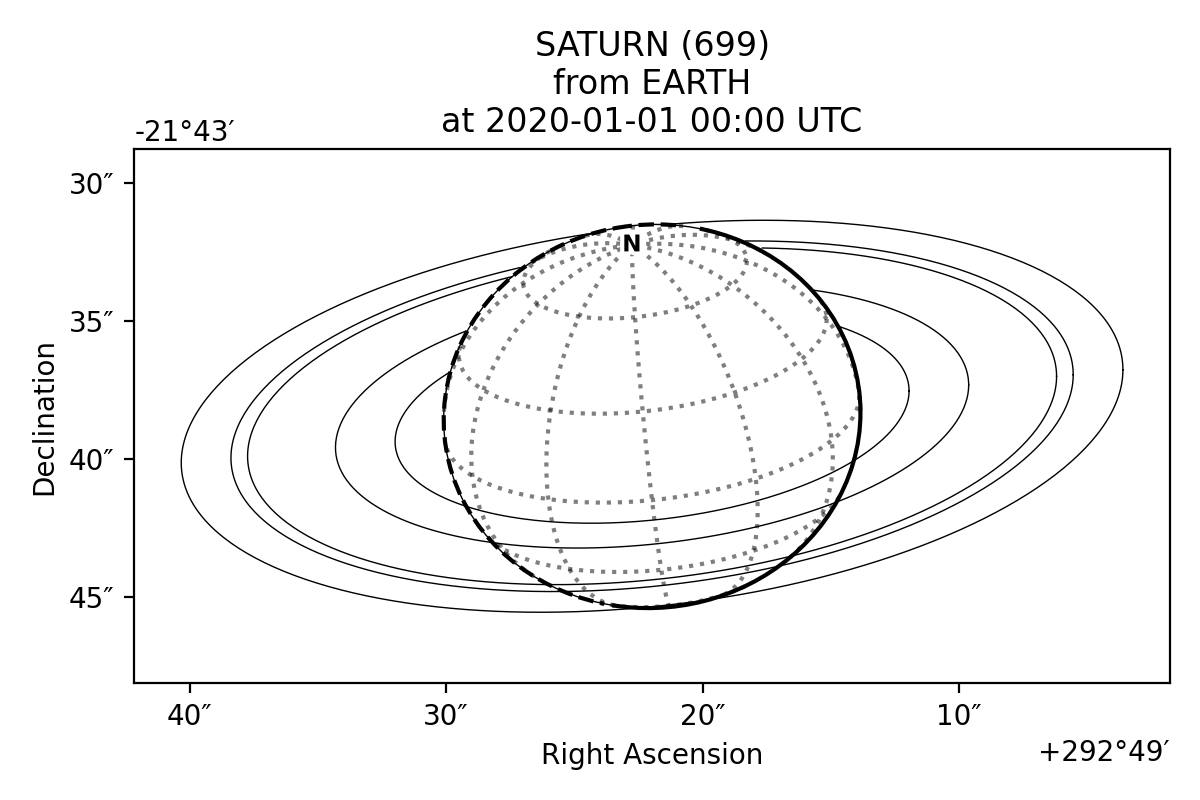More complex plots can also be created using the functionality in `planetmapper.Body` and manually adding elements to the plot:

```body = planetmapper.Body('neptune', '2020-01-01')

# Add Triton to any wireframe plots

# Mark this specific coordinate (if visible) on any wireframe plots
body.coordinates_of_interest_lonlat.append((360, -45))

# Add Neptune's rings to the plot

fig, ax = plt.subplots(figsize=(6, 6), dpi=200)

# Manually add some text to the plot
ax.text(
body.target_ra, body.target_dec + 2 / 60 / 60, 'NEPTUNE', color='b', ha='center'
)

plt.show()
```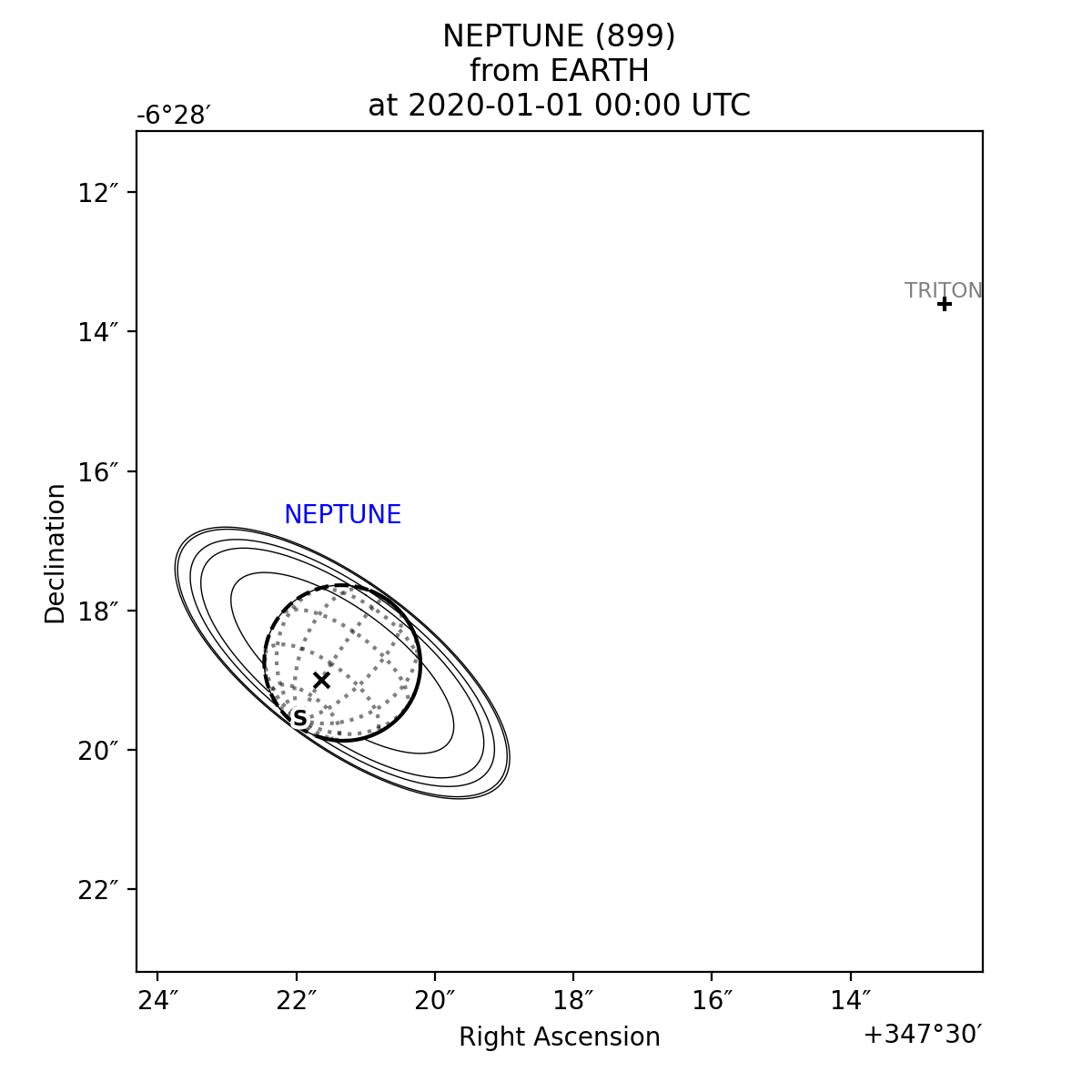A number of different wireframe plotting options are available:

`plot_wireframe_km` is particularly useful for comparing observations taken at different times, as it standardises the position, orientation and size of the target body. The example below shows multiple observations of Jupiter and Io taken over the space of a few hours. Jupiter moves across the the RA/Dec plot (top), but stays fixed in the km plot (bottom), making it easier to see the relative motion of Io:

```fig, [ax_radec, ax_km] = plt.subplots(nrows=2, figsize=(6, 8), dpi=200)

dates = ['2020-01-01 00:00', '2020-01-01 01:00', '2020-01-01 02:00']
colors = ['r', 'g', 'b']

for date, c in zip(dates, colors):
body = planetmapper.Body('jupiter', date)
body.plot_wireframe_km(ax_km, color=c)

# Plot some blank data with the correct colour to go on the legend

ax_km.set_title('Position relative to Jupiter')

fig.tight_layout()
plt.show()
```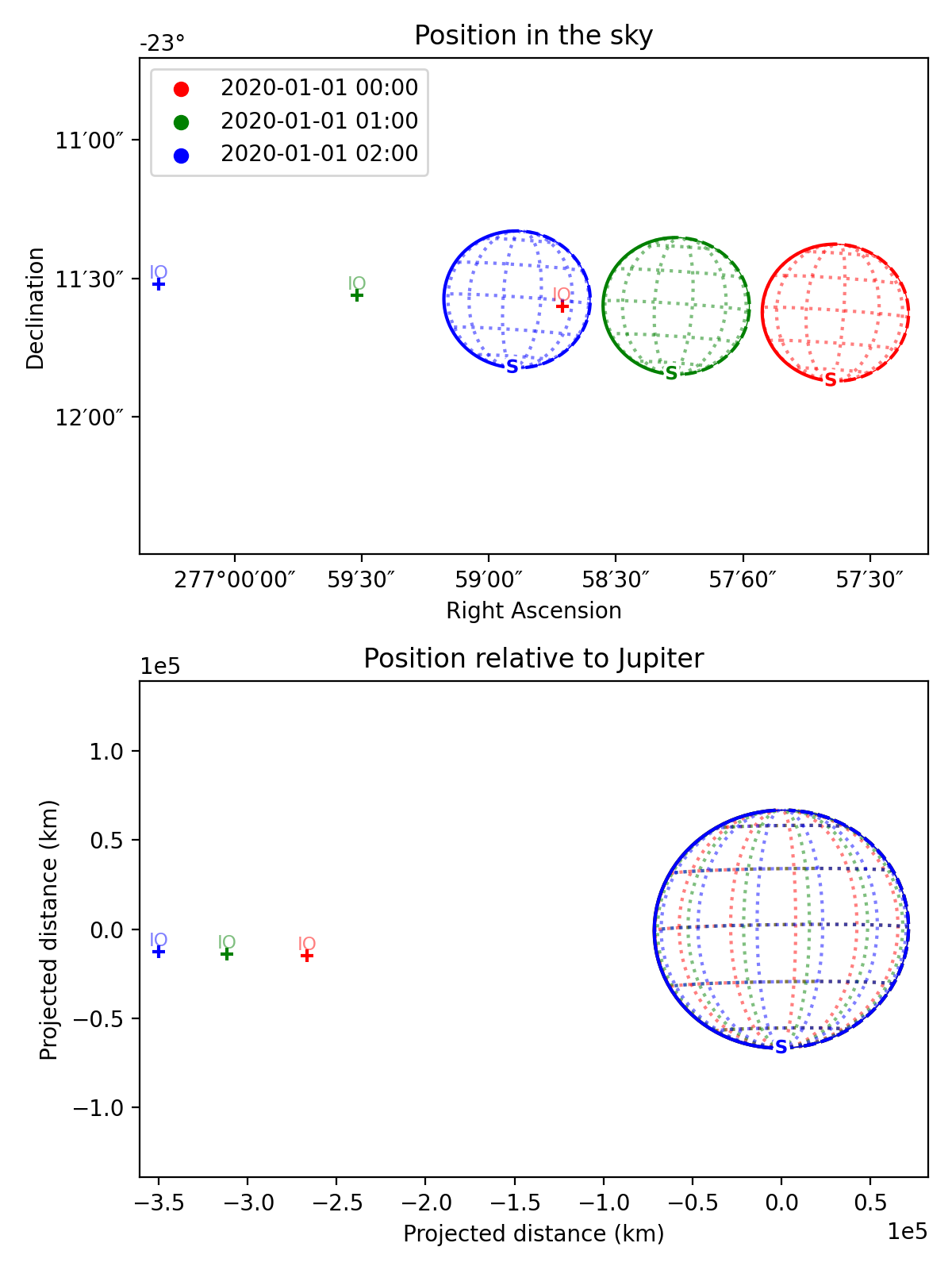## Observations, backplanes and mapping

`planetmapper.Observation` objects can be created to calculate information about a specific observation. If the observed data is saved in a FITS file with appropriate header information, a `planetmapper.Observation` object can be created using only the path to that file - target, date and observer information can all be derived automatically from the header. The example below creates an Observation object, and uses it to plot an image containing showing the longitude value of each pixel:

```observation = planetmapper.Observation('../data/europa.fits.gz')

# Set the disc position
observation.set_plate_scale_arcsec(12.25e-3)
observation.set_disc_params(x0=110, y0=104)

observation.plot_backplane_img('LON-GRAPHIC')
plt.show()
```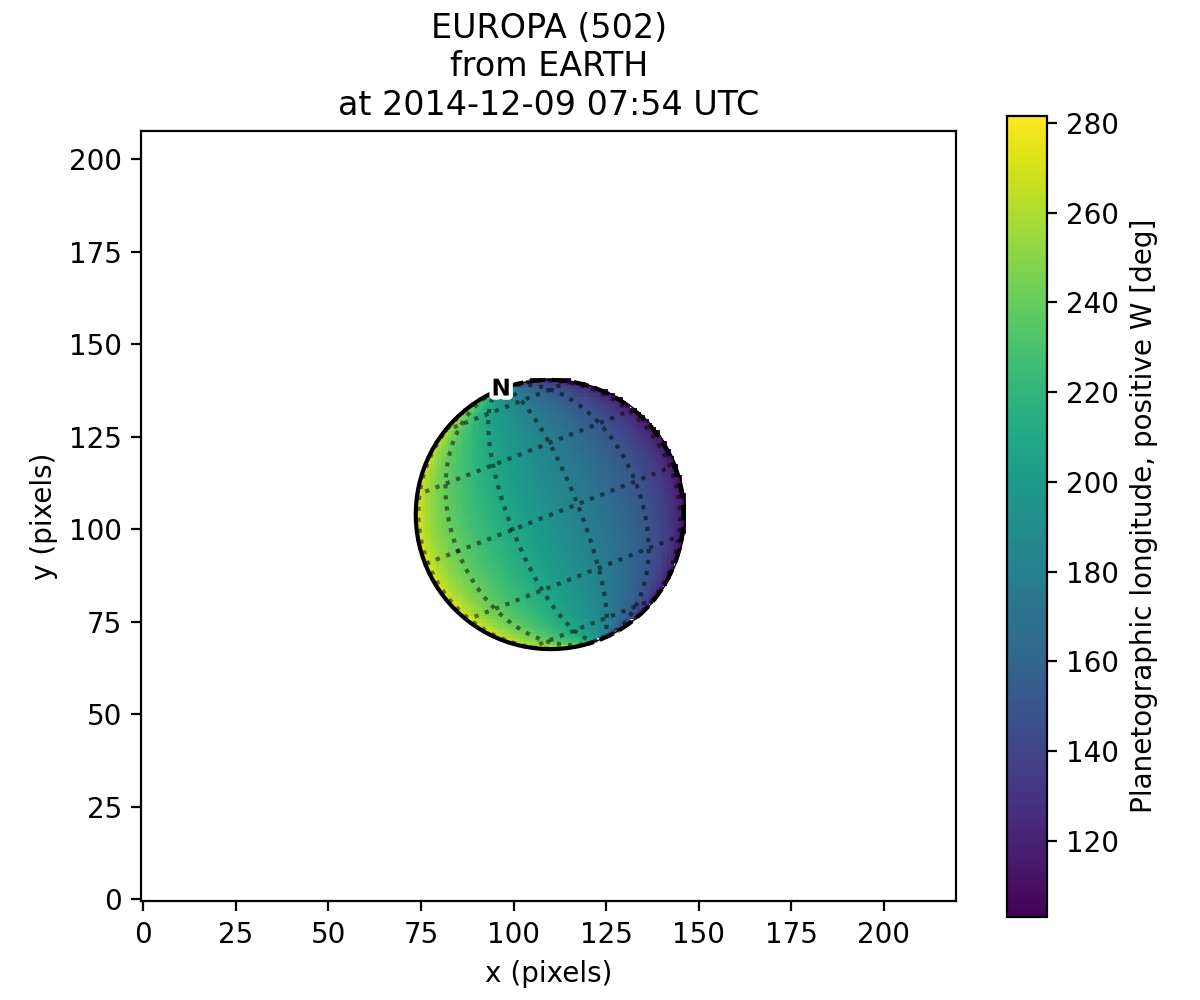A range of backplane images can be generated - see Default backplanes for a list of the backplanes available by default. These backplanes can be saved to a FITS file for future use using `planetmapper.Observation.save_observation()`. A mapped version of the image and backplanes can likewise be saved using `planetmapper.Observation.save_mapped_observation()`:

```observation = planetmapper.Observation('../data/europa.fits.gz')

# Set the disc position
observation.set_plate_scale_arcsec(12.25e-3)
observation.set_disc_params(x0=110, y0=104)

observation.save_observation('europa_navigated.fits')
observation.save_mapped_observation('europa_mapped.fits')
```

Mapped data can also be manipulated and plotted directly. In the example below, we use `planetmapper.Observation.get_mapped_data()` and `planetmapper.BodyXY.get_backplane_map()` to directly access, manipulate and plot the mapped data and backplanes:

```# This uses a JPG image, so we need to manually specify details (e.g. target)
observation = planetmapper.Observation(
'../data/jupiter.jpg',
target='jupiter',
utc='2020-08-25 02:30:40',
observer='HST',
show_progress=True, # show progress bars for slower functions
)

# Run the GUI to fit the disc interactively
observation.run_gui()

fig, axs = plt.subplots(
nrows=2, ncols=2, figsize=(12, 8), dpi=200, width_ratios=[1, 2]
)

# Do a nice RGB plot of the data in the top left
rgb_img = np.moveaxis(observation.data, 0, 2)  # imshow needs wavelength index last
axs[0, 0].imshow(rgb_img, origin='lower')
observation.plot_wireframe_xy(axs[0, 0])

# Plot the emission angle backplane in the bottom left
observation.add_other_bodies_of_interest('Europa')  # mark Europa on this plot
observation.plot_backplane_img('EMISSION', ax=axs[1, 0])

# Plot the mapped emission angle backplane in the bottom right
observation.plot_backplane_map('EMISSION', ax=axs[1, 1])

# Plot a mapped RGB image of the data in the top right
degree_interval = 0.25  # Plot maps with 4 pixels/degree
emission_cutoff = 80

mapped_data = observation.get_mapped_data(degree_interval)  # get the mapped data
rgb_map = np.moveaxis(mapped_data, 0, 2)  # imshow needs wavelength index last
rgb_map = planetmapper.utils.normalise(rgb_map)  # normalise to make plot look nicer

# Only plot areas with emission angles <80deg
emission_map = observation.get_backplane_map('EMISSION', degree_interval)
for idx in range(3):
rgb_map[:, :, idx][np.where(emission_map > emission_cutoff)] = 1

# Display mapped image and add a useful annotation
observation.imshow_map(rgb_map, ax=axs[0, 1])
axs[0, 1].annotate(
f'Showing emission angles < {emission_cutoff}°',
(0.005, 0.99),
xycoords='axes fraction',
size='small',
va='top',
)

for ax in axs.ravel():
ax.set_title('')
fig.suptitle(observation.get_description(multiline=False))
fig.tight_layout()

plt.show()
```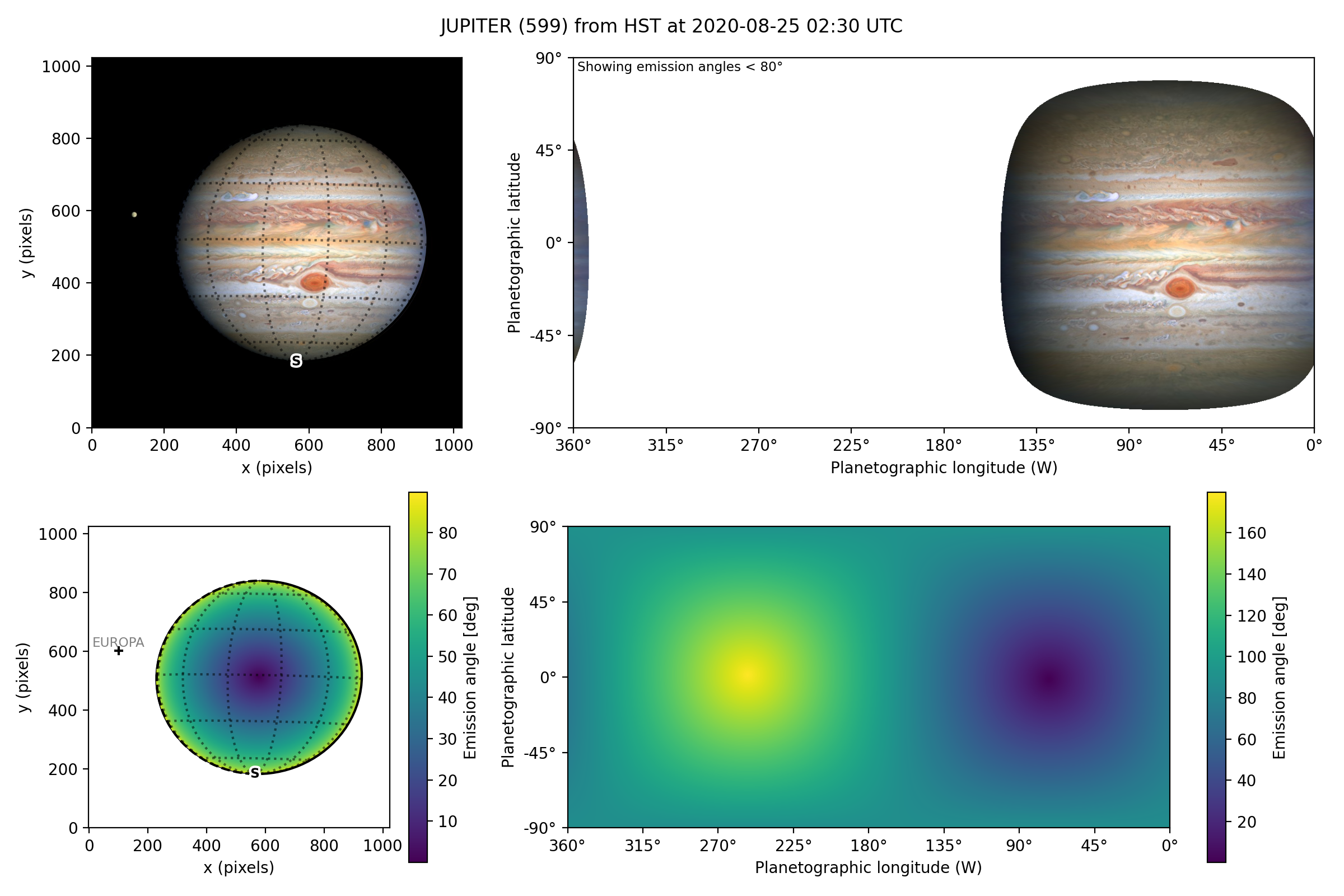Backplanes can also be generated for observations which do not exist using `planetmapper.BodyXY`:

```# Create an object representing how Jupiter would appear in a 50x50 pixel image
# taken by JWST at a specific time
body = planetmapper.BodyXY('jupiter', utc='2024-01-01', observer='JWST', sz=50)
body.set_disc_params(x0=25, y0=25, r0=20)

fig, ax = plt.subplots(figsize=(6, 5), dpi=200)
fig.tight_layout()
plt.show()

# Backplane images can also be accessed and manipulated directly

# Average radial velocity: 25.27 km/s
```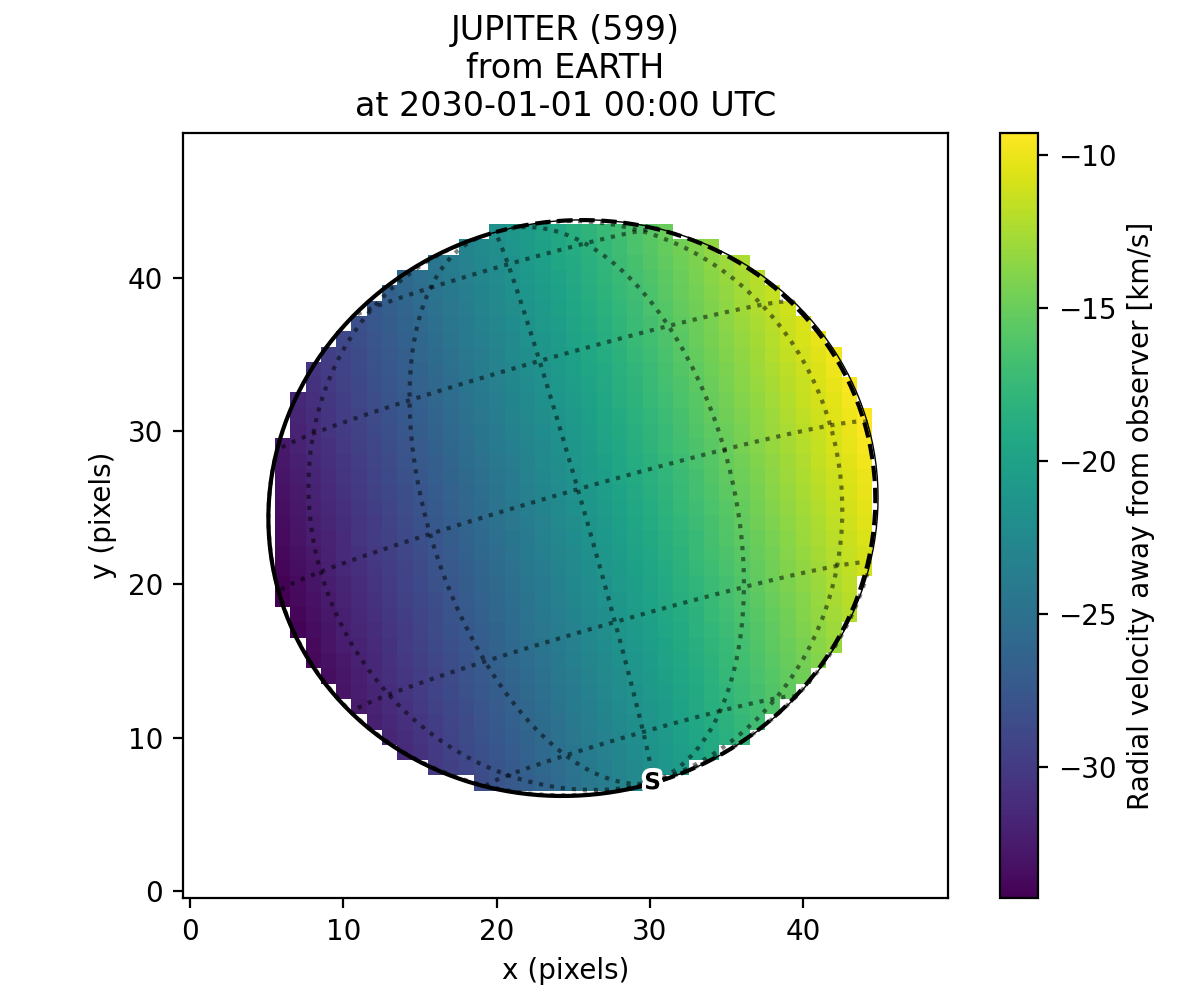## Cache behaviour

The generation of backplanes and projected mapped data can be slow for larger datasets. Therefore, `planetmapper.BodyXY` and `planetmapper.Observation` objects automatically cache the results of various expensive function calls so that they do not have to be recalculated. This cache management happens automatically behind the scenes, so you should never have to worry about dealing with it directly. For example, when any disc parameters are changed, the cache is automatically cleared as the cached results will no longer be valid.

```# Create a new object
body = planetmapper.BodyXY('Jupiter', '2000-01-01', sz=500)
body.set_disc_params(x0=250, y0=250, r0=200)
# At this point, the cache is completely empty

# The intermediate results used in generating the incidence angle backplane
# are cached, speeding up any future calculations which use these
# intermediate results:
body.get_backplane_img('INCIDENCE') # Takes ~10s to execute
body.get_backplane_img('INCIDENCE') # Executes instantly
body.get_backplane_img('EMISSION') # Executes instantly

# When any of the disc parameters are changed, the xy <-> radec conversion
# changes so the cache is automatically cleared (as the cached intermediate
# results are no longer valid):
body.set_r0(190) # This automatically clears the cache
body.get_backplane_img('EMISSION') # Takes ~10s to execute
body.get_backplane_img('INCIDENCE') # Executes instantly
```

The methods which cache their results include…

Note

The Python script used to generate all the figures shown on this page can be found here